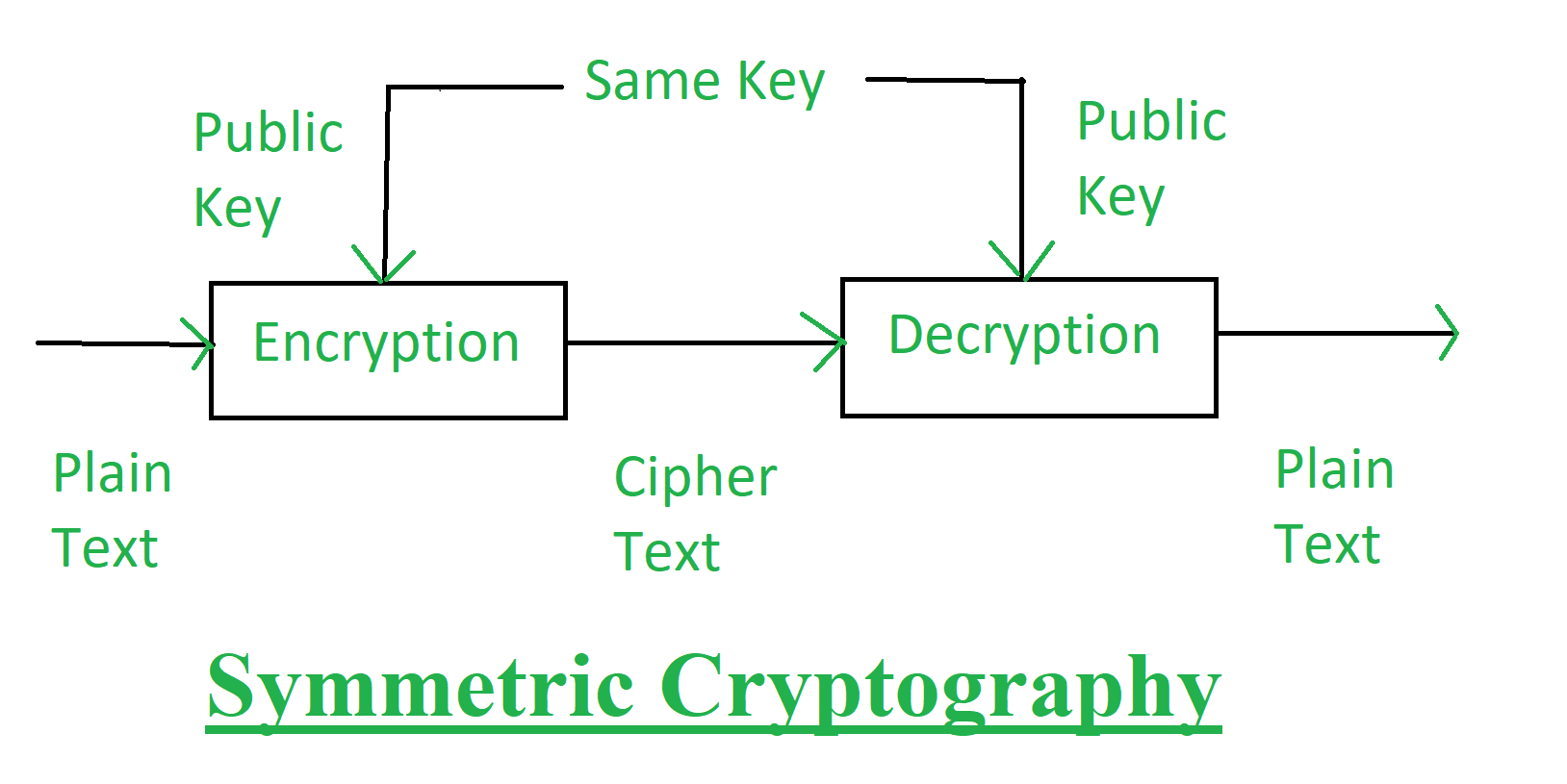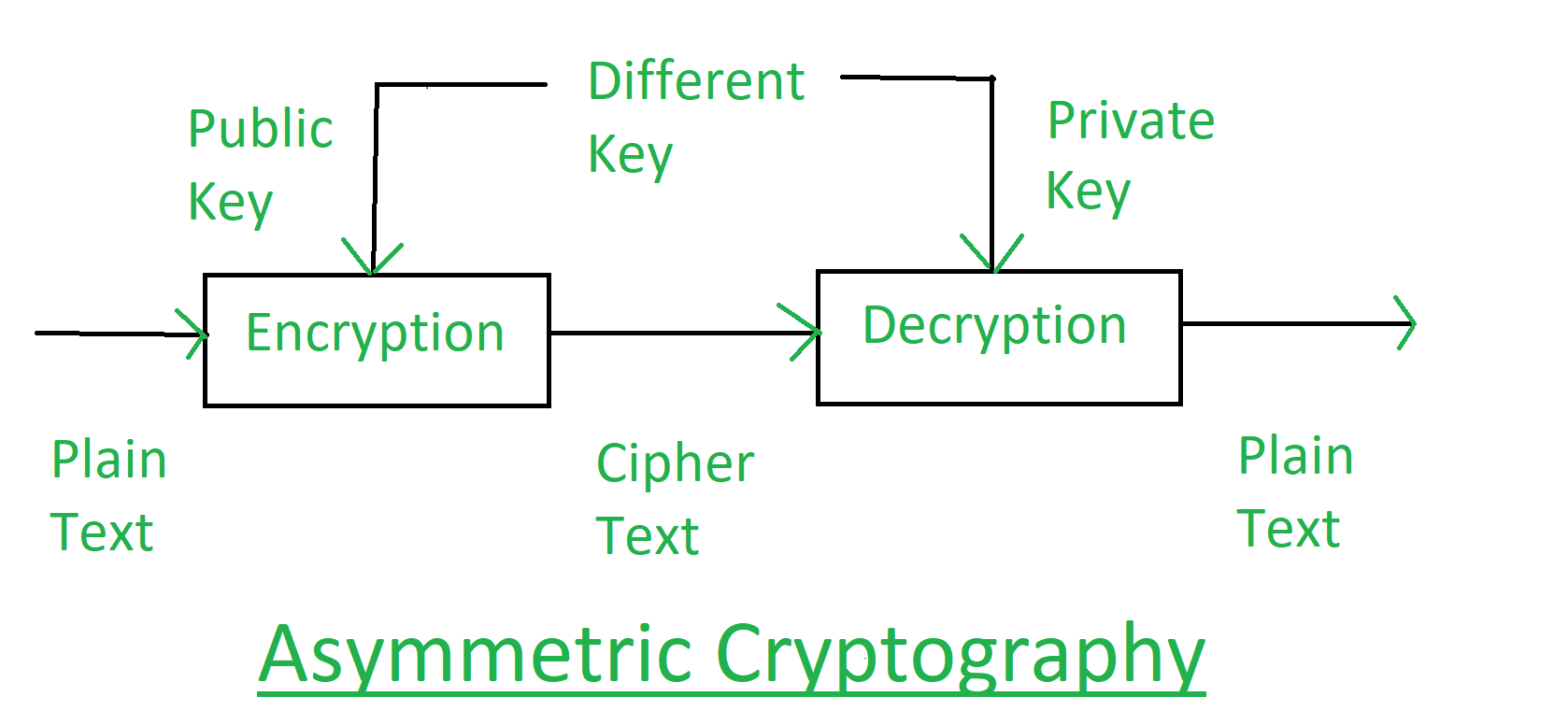Related Articles

# Classical Cryptography and Quantum Cryptography

• Last Updated : 08 Aug, 2019

Cryptography is the technique which is used for doing secure communication between two parties in the public environment where unauthorized users and malicious attackers are present. In cryptography there are two processes i.e. encryption and decryption performed at sender and receiver end respectively. Encryption is the processes where a simple multimedia data is combined with some additional data (known as key) and converted into unreadable encoded format known as Cipher. Decryption is the reverse method as that of encryption where the same or different additional data (key) is used to decode the cipher and it is converted in to the real multimedia data.

Cryptography techniques can be categorized according to their basic principles or protocols they follow. But here we are going to concentrate on the two types of cryptography technique: Classical Cryptography and Quantum Cryptography. These are explained as following below.

1. Classical Cryptography:
Classical cryptography is based on the mathematics and it relies on the computational difficulty of factorizing large number. The security of classical cryptography is based on the high complexity of the mathematical problem for the instance factorization of large number.

In the classical cryptography the original data i.e., the plain text is transformed into the encoded format i.e. cipher text so that we can transmit this data through insecure communication channels. A data string which known as key is used to control the transformation of the data from plain text to cipher text. This arrangement helps to keep data safe as it required the key for extracting the original information from the cipher text. Without the key no one can read the data. In this technique it is assumed that the only authorized receiver has the key.

Classical Cryptography has two types of techniques:

1. Symmetric Cryptography:
In the symmetric cryptography a single key is used for encrypting and decryption the data. This encryption key is private key. This is the limitation of this encryption technique that this private key must be distributed only among the authorized sender and receiver.2. Asymmetric Cryptography:
In the asymmetric cryptography a pair of key, i.e., public key and private key is used for encryption and decryption. A sender can use its public key to encrypt the data and on receiver end receiver can decrypt the data by using its private key. This technique overcomes the problem of key distribution.• While employing the one-time pad, it is unbreakable.
• It is easy to do manually, no computer required.
• It protects the plain text from casual snooping.

• While employing the one-time pad, it is cumbersome and requires a personal meetup to exchange the pads.
• If not employing the OTP, anyone who is even remotely interested in knowing what you wrote and knows about cryptography will be able to break the encryption.

2. Quantum Cryptography:
Quantum Cryptography is based on physics and it relies on the laws of quantum mechanics. It is arising technology which emphasizes the phenomena of quantum physics in which two parties can have secure communication based on the invariabilities of the laws of the quantum mechanics. Quantum mechanics is the mathematical framework or set of rules for the construction of physical theories.

There are 2 important elements of quantum mechanics on which quantum cryptography depends: Heisenberg Uncertainty Principle and Photon Polarization Principle. These are explained as following below.

1. Heisenberg Uncertainty Principle:
This principle says that if you measure one thing, you cannot measure another thing accurately. For example, if you apply this principle to human, you could measure a person’s height, but you can’t measure his weight. The only odd thing about this principle is that it becomes true only for the instant at which you try to measure something. This principle is applied to the photons. Photons have wave like structure and are polarized or tilted in certain direction. While measuring photon polarization, all subsequent measurements are get affected by the choice of measures that we made for polarization. This principle plays the vital role to prevent the efforts of attacker in quantum cryptography.
2. Photon Polarization Principle:
This principle refers that, an eavesdropper cannot copy the unique quantum bits, i.e., unknown quantum state, due to the no-cloning principle. If an attempt is made for measuring any properties, it will disturb the other information.

• It establishes secure communication by providing security based on fundamental laws of physics instead of mathematical algorithms or computing technologies used today.
• It is virtually unhackable.
• It is simple to use.
• Less resources are needed in order to maintain it.
• It is used to detect eavesdropping in QKD (Quantum Key Distribution). This is due to the fact that it is not possible to copy the data encoded in quantum state.
• The performance of such cryptography systems is continuously improved.